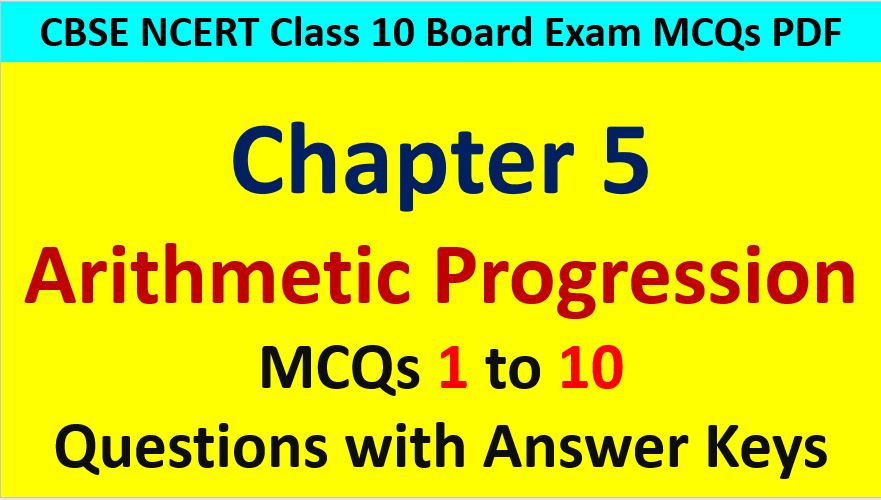Friday, October 22, 2021
Home > CBSE Class 10 > MCQ Questions for Class 10 Maths Chapter 5 Arithmetic Progression with Answer Keys

# MCQ Questions for Class 10 Maths Chapter 5 Arithmetic Progression with Answer KeysHi students, Welcome to AMBiPi (Amans Maths Blogs). In this article, you will get MCQ Questions for Class 10 Maths Chapter 5 Arithmetic Progression with Answer Keys PDF. You can download this PDF and save it in your mobile device or laptop etc.

MCQ Questions for Class 10 Maths Chapter 5 Arithmetic Progression Question No 1:

The nth term of the AP a , 3a , 5a , … is

Option A : na

Option B : (2n – 1)a

Option C : (2n + 1)a

Option D : 2na

Option B : (2n – 1)a

MCQ Questions for Class 10 Maths Chapter 5 Arithmetic Progression Question No 2:

The number of terms common to the two A.P. s 2 + 5 + 8 + 11 + ….+ 98 and 3 + 8 + 13 + 18 +….+198 is

Option A : 33

Option B : 40

Option C : 4

Option D : None of These

Option C : 7

MCQ Questions for Class 10 Maths Chapter 5 Arithmetic Progression Question No 3:

The common difference of the AP 1/p, (1-p)/p, (1-2p)/p, is

Option A : 1

Option B : 1/p

Option C : -1

Option D : -1/p

Option C : -1

MCQ Questions for Class 10 Maths Chapter 5 Arithmetic Progression Question No 4:

How many terms of AP 54, 51, 48… are required to give a sum of 513?

Option A : 21 or 23

Option B : 22 or 23

Option C : 23 or 24

Option D : 18 or 19

Option D : 18 or 19

MCQ Questions for Class 10 Maths Chapter 5 Arithmetic Progression Question No 5:

Find the next two terms of the A.P.: -10, -6, -2, …

Option A : 4 and 8

Option B : -4 and -8

Option C : 2 and 6

Option D : 6 and 10

Option C : 2 and 6

MCQ Questions for Class 10 Maths Chapter 5 Arithmetic Progression Question No 6:

The value of x for which 2x, (x + 10) and (3x + 2) are the three consecutive terms of an AP, is

Option A : 6

Option B : -6

Option C : 18

Option D : -18

Option A : 6

MCQ Questions for Class 10 Maths Chapter 5 Arithmetic Progression Question No 7:

How many terms are there in 20, 25, 30………, 140?

Option A : 22

Option B : 23

Option C : 24

Option D : 25

Option B : 25

MCQ Questions for Class 10 Maths Chapter 5 Arithmetic Progression Question No 8:

The first term of AP is p and the common difference is q , then its 10th term is

Option A : q + 9p

Option B : p – 9q

Option C : p + 9q

Option D : 2p + 9q

Option C : p + 9q

MCQ Questions for Class 10 Maths Chapter 5 Arithmetic Progression Question No 9:

The fourth term of an A.P. is 4. Then the sum of the first 7 terms is :

Option A : 4

Option B : 28

Option C : 16

Option D : 40

Option B : 28

MCQ Questions for Class 10 Maths Chapter 5 Arithmetic Progression Question No 10:

In an AP, if d =− 4, n = 7 and an = 4, then a is equal to

Option A : 6

Option B : 7

Option C : 20

Option D : 28